# How to convert dates to month, year and day in excel?

July 22, 2022
2.7K Views
0

A free Office suite fully compatible with Microsoft OfficeThere may be times while working with date-specific data that you need to identify the weekday, month, or year that corresponds to a certain date. For example, you might be interested in learning the day of the week instead than just the date or you want to convert date to month.

Excel automatically detects the format and changes any date you enter in cell into a date cell. Excel is thus aware of which parts of the date you supplied correspond to the month, the year, and the day.

This has a lot of potential benefits. One special advantage of this ability of excel is that it enables you to extract the desired parts of the date. In this article, we will look at three distinct Excel date conversion methods.

# Convert date to year in excel

This method use the YEAR function to convert date to year in excel. Here is the step by step tutorial on how you can do it.

1. Write your sample dates in a column.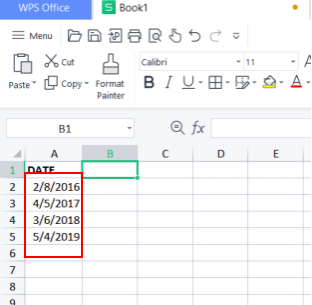1. Type the formula: =YEAR(A2), where A2 represent number of cell.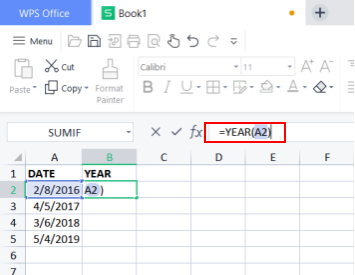1. Press the return key.

2. This should display the year.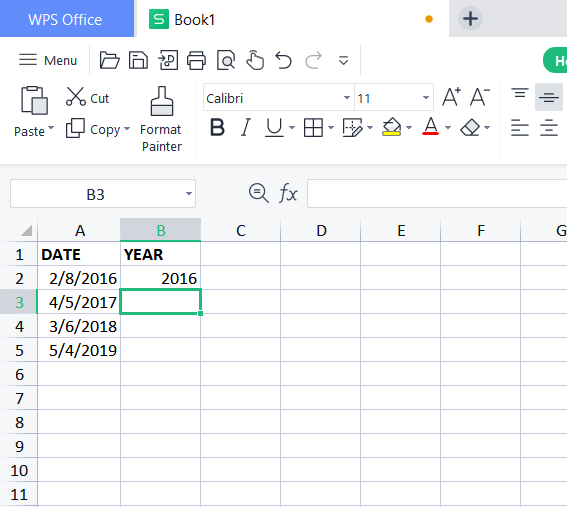1. Follow the above method with all the dates, just changing the name of corresponding cell in the formula or use the Fill Handle that will auto fill the lower cells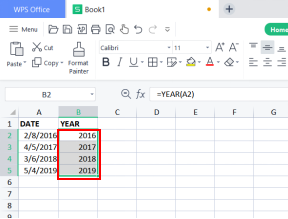# Converting date to month in excel

This method use the MONTH function to convert date to month in excel. Here is the step by step tutorial on how you can convert date to month in excel.

1. Write your sample dates in a column.

2. Type the formula: =MONTH(A2), where A2 represent number of cell.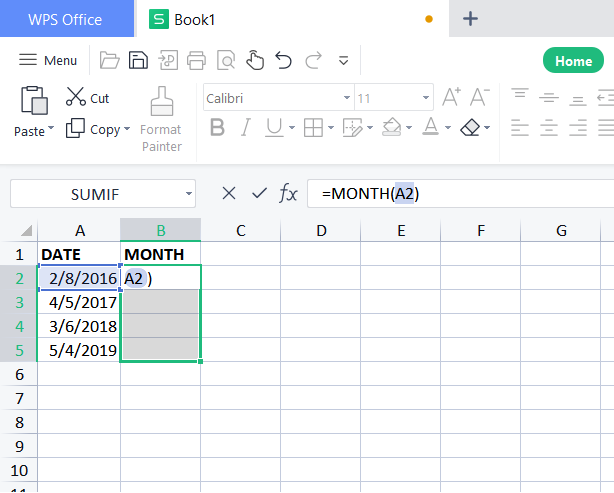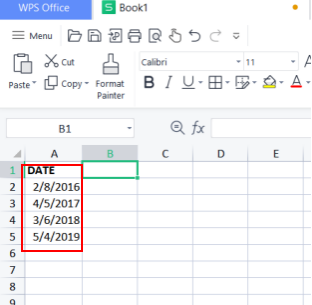1. Press the return key.

2. This should convert the date to month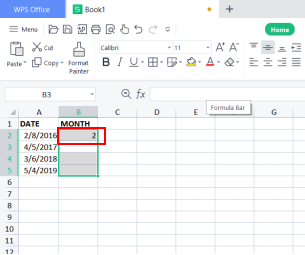1. Follow the above method with all the dates, just changing the name of corresponding cell in the formula or use the Fill Handle that will auto fill the lower cells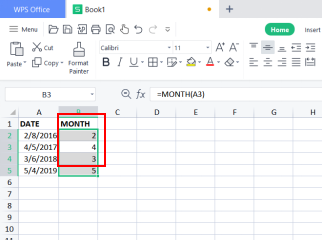# Converting date to day in excel

We can convert date to day with TEXT function. Here is the stepwise tutorial.

1. Write sample dates in a column.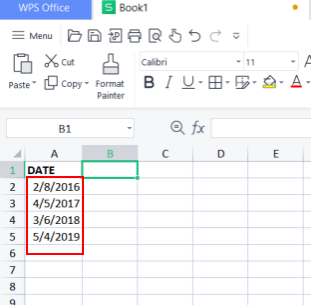1. Click on the cell where you want the day to be displayed.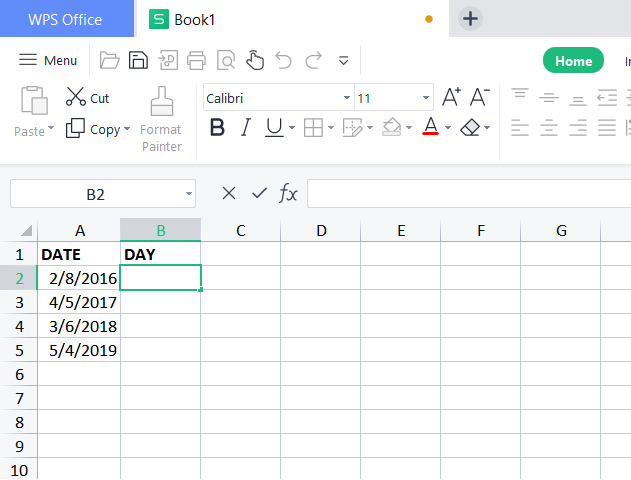1. Type the formula: =TEXT(A2,”ddd”), where A2 represent the cell number. You can also use “dddd” for displaying full name of day.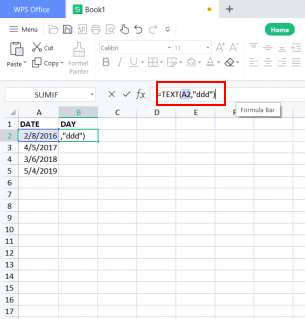1. Press the return key

2. it should display the name of the day.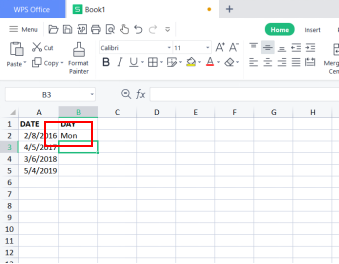1. Now you can type the name of the cells separately in function or use the Fill handle to auto fill the rest of the cells.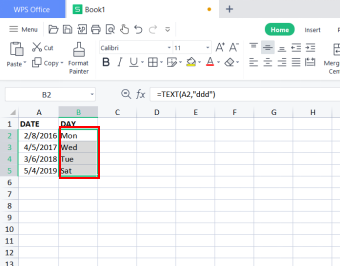The above tutorials are usable in WPS 2016/2019 versions. WPS can be used across various platforms such as windows, mac, iPhone, etc. So, you can use these tutorials on any device without being online.